# [CVPR 2023 Series #3] GENIE: Show Me the Data for Quantization

By Yongkweon Jeon Samsung Research
By Chungman Lee Samsung Research
By Ho-young Kim Samsung Research
 The Computer Vision and Pattern Recognition Conference (CVPR) is a world-renowned international Artificial Intelligence (AI) conference co-hosted by the Institute of Electrical and Electronics Engineers (IEEE) and the Computer Vision Foundation (CVF) which has been running since 1983. CVPR is widely considered to be one of the three most significant international conferences in the field of computer vision, alongside the International Conference on Computer Vision (ICCV) and the European Conference on Computer Vision (ECCV). In this relay series, we are introducing a summary of the 6 research papers at the CVPR 2023 and here is a summary of them. - Part 1 : SPIn-NeRF: Multiview Segmentation and Perceptual Inpainting with Neural Radiance Fields (by Samsung AI Center – Toronto) - Part 2 : StepFormer: Self-supervised Step Discovery and Localization in Instructional Videos (by Samsung AI Center – Toronto) - Part 3 : GENIE: Show Me the Data for Quantization (by Samsung Research) - Part 4 : A Unified Pyramid Recurrent Network for Video Frame Interpolation(by Samsung R&D Institute - Nanjing) - Part 6 : LASP: Text-to-Text Optimization for Language-Aware Soft Prompting of Vision & Language Models (By Samsung AI Center - Cambridge) - Part 7 : Zero-Shot Everything Sketch-Based Image Retrieval, and in Explainable Style (By Samsung AI Center - Cambridge)

## Introduction

Since the scale of the state-of-the-art AI models has become deeper, model compression also has been attracting more attention as a method to let models be deployed on edge devices without accessing cloud servers. Among the various approaches for compressing models, quantization is a very promising scheme in terms of reducing the model size and accelerating inference. By representing tensors using a lower bit width and maintaining a dense format of tensors, quantization reduces a computing unit to a significantly smaller size compared to that achieved by other approaches (such as pruning and low-rank approximations) and facilitates massive data parallelism with vector processing units.

Recent studies have emphasized post-training quantization (PTQ) [5, 7, 9] because it serves as a convenient method of producing high-quality quantized networks with only a small amount of unlabeled datasets or even in the absence of a dataset (including synthetic datasets). Because PTQ can compress models within a few hours but shows comparable performance to quantization-aware training (QAT) based on retraining, PTQ is preferred over QAT in practical situations.

According to the given condition with respect to the dataset, post-training quantization can be classified into two categories: Few-shot quantization (FSQ) compresses the models with a small amount of unlabeled dataset for calibration while Zero-shot quantization (ZSQ) aims to reduce the accuracy drop without employing real datasets when compressing models. ZSQ thus performs the additional process to generate elaborate replicas being replaced with the real.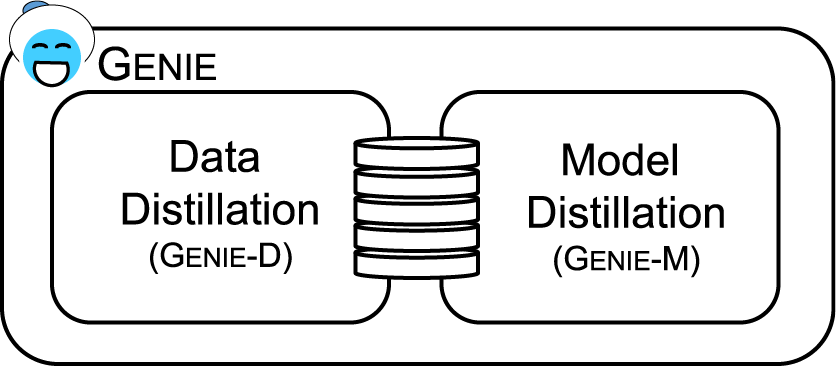Figure 1.  Conceptual illustration of GENIE, which consists of two sub-modules: synthesizing data and quantizing models

In this blog, we introduce a framework called GENIE that efficiently quantizes models regardless of whether the given data is small (Few-shot) or even absent (Zero-shot). GENIE is made up of two sub-modules: GENIE-D and GENIE-M (see Figure 1). Given zero-shot, data distillation (or generation) is followed by model distillation for quantization, while the process of distilling data can be omitted given few-shot.

## Data Distillation (GENIE-D)

We synthesize or distill dataset x by model inversion , which is to learn the data (utilized in a pre-training stage) instead of the parameters. In other words, we can find (or optimize) x maximizing the below probability:where fθ is a pre-trained model with leaned parameter θ. To be more specific, we set the loss to distill the data x as follows:whereand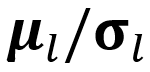represent the statistical parameters of the synthetic data and learned parameters in the l-th batch normalization layer, respectively. We utilize statistics (mean µ and standard deviation σ) in the batch normalization layers (BNS) of the pre- trained models to distill data x.

Based on the above, we design a generator that produces synthetic data but distills the knowledge to latent vectors z from a normal distribution. In other words, the synthetic images are distilled indirectly by the latent vectors which are trained and updated in every iteration.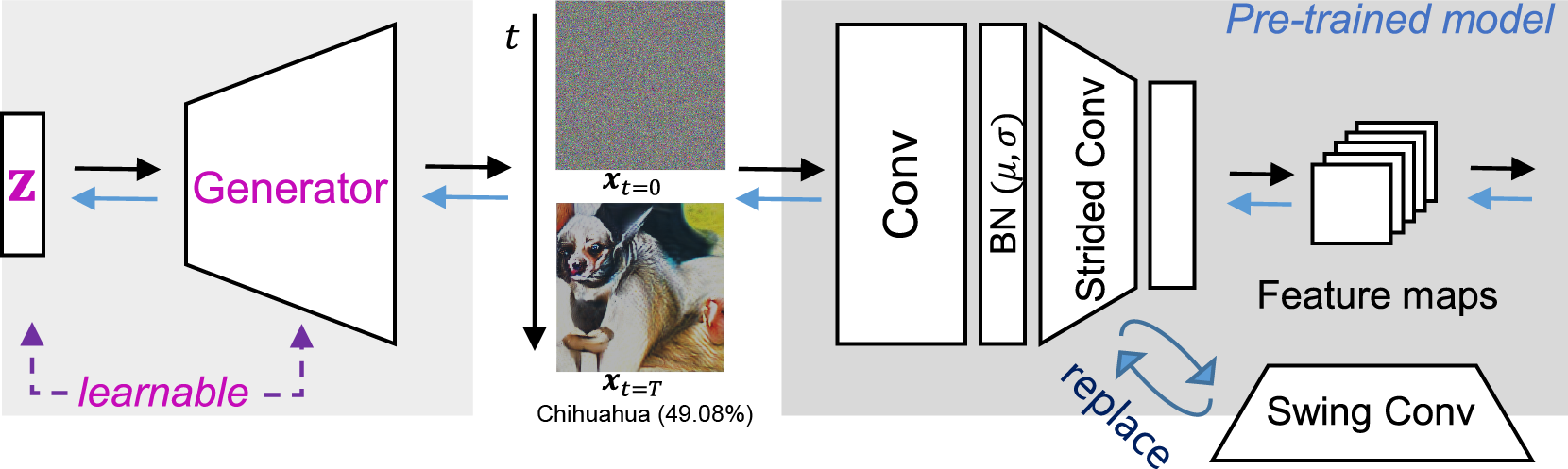Figure 2.  Data distillation (from noise (t = 0) to signal (t = T)). We train the generator and latent vectors z, each of which is a kind of seed that generates its own image. The synthetic dataset is distilled indirectly by learning the latent vectors and the generator. When distilling images, n(> 1)stride convolutions on pre-trained models are replaced by swing convolutions.

Figure 2 illustrates the proposed method for distilling datasets. The latent vector initialized in the Gaussian form becomes an image via the generator, and the image takes the loss from the pre-trained model; the latent vector and generator are updated by the loss. The images from the initial vectors are close to noise, but they gradually mature into information suited for quantization as the optimization goes on. The image indicated by xt=T in Figure 2 is a distilled image updated by the BNS loss (Eq. 2) and swing convolution (Figure 3) without any image prior loss. By optimizing in manifold space (latent vector) (n-dimension) rather than optimizing in pixel space (m-dim, nm), GENIE can efficiently explore attributes of the real images pre-trained models utilized while training the generator for common knowledge or image prior.

## Swing Convolution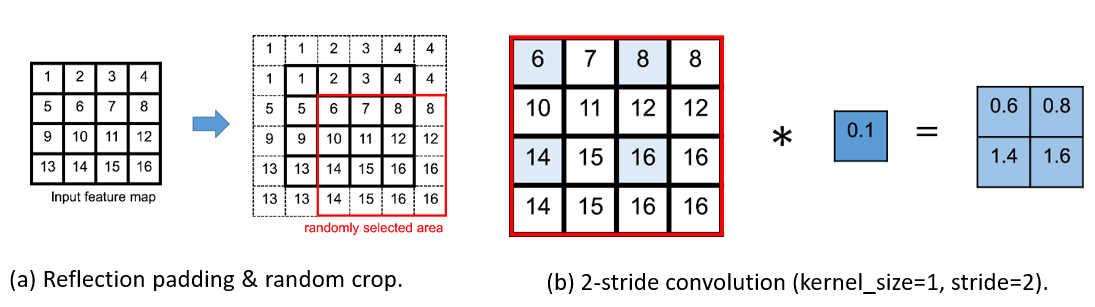Figure 3.  Swing convolution. (a) feature maps are extended by reflection padding and randomly cropped (b) The randomly selected areas in feature maps are convolved with the stride of n (n > 1).

When performing conv of stride n (n > 1), it causes information loss because pixels of fixed rows and columns in feature maps (not all pixels) are utilized, which can result in generating lower-quality of data during data distillation . Suppose that there is a 1 × 1 convolutional layer of stride 2 with 4 × 4 feature maps as input (see Figure 3b) for example, pixels in the second and fourth row and columns are not utilized and thus not used for back-propagation. In other words, the information on the pixels not used is lost.

To head off this issue, we introduce swing convolution performing stochastic n-stride conv, which is simple but effective in preventing information loss in the convolution of the stride n (n > 1). With the reduction of information loss, distilled images become considerably robust and then enhance the quality of quantized models.

Figure 3 illustrates the mechanism of swing conv. Before 2-stride conv, the feature maps are extended by padding with their edge values (i.e.,, reflection padding) and randomly cropped to restore them to their original sizes, as shown in Figure 3a. Then, the randomly selected areas in the feature maps are stride-convolved as shown in Figure 3b. We refer to this series of processes as swing conv. We replace all s-convs to swing convs when only synthesizing the datasets. By applying randomness to the feature maps to be convolved, the distilled images can be updated so that the statistics of the outputs match the BNS regardless of the feature maps selected randomly.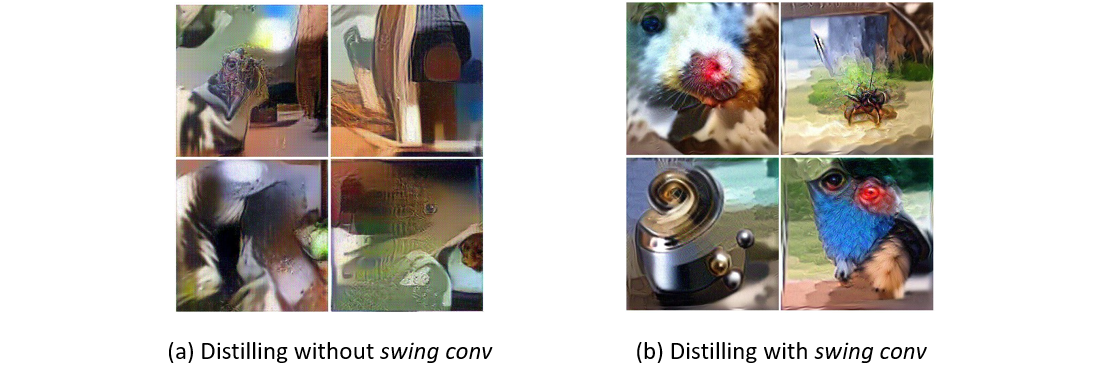Figure 4.  The effect of swing convolution that reduces information loss. The images in the same cell in each grid were distilled from the same seed. The images were directly distilled without the generator.

## Quantization (GENIE-M)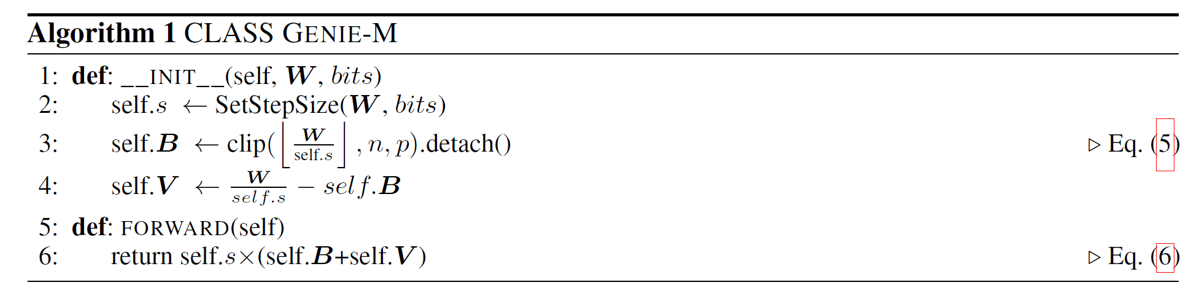Supposing the step size s ∈ R is set by a certain algorithm, Wint and Wq can be defined as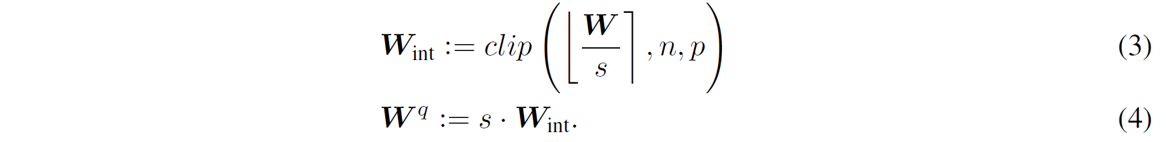where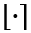denotes the nearest-rounding method, and n and p represent the lower and upper bound of the range, respectively. Let the base integer matrix B[n,p]m×n be defined aswhere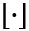denotes the floor function. Then, AdaRound  sets the quantized weights W q as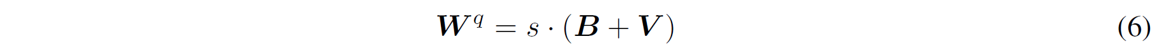where V ∈ [0,1]m×n is the softbit matrix to be optimized such that each weight wint,i (∈ Wint) is converged to either bi (∈ B) or bi + 1. However, it can cause a conflict with the softbits V being optimized when optimizing s in addition to V, because optimizing the step size s results in the change in the base B. To resolve this issue, we suggest a method to enable joint optimization without conflict via a sub-module of GENIE (GENIE-M). Regardless of the s being optimized, we maintain B as initialized by releasing the mutual dependency between B and s. B is considered constant and not dependent on s, and the losses propagated to s, v (∈ V ), and b (∈ B) during optimization are computed as follows:Algorithm 1 and 2 present the pseudo-code for GENIE-M, which is the sub-module used for distilling models in GENIE. Using this quantizer, we optimize the quantized models by minimizing the blockwise reconstruction error, like that in BRECQ . GENIE-M also uses LSQ  to optimize the step size of activations, which can be combined with QDROP . All activations in a block are simultaneously optimized with all the weights in the block.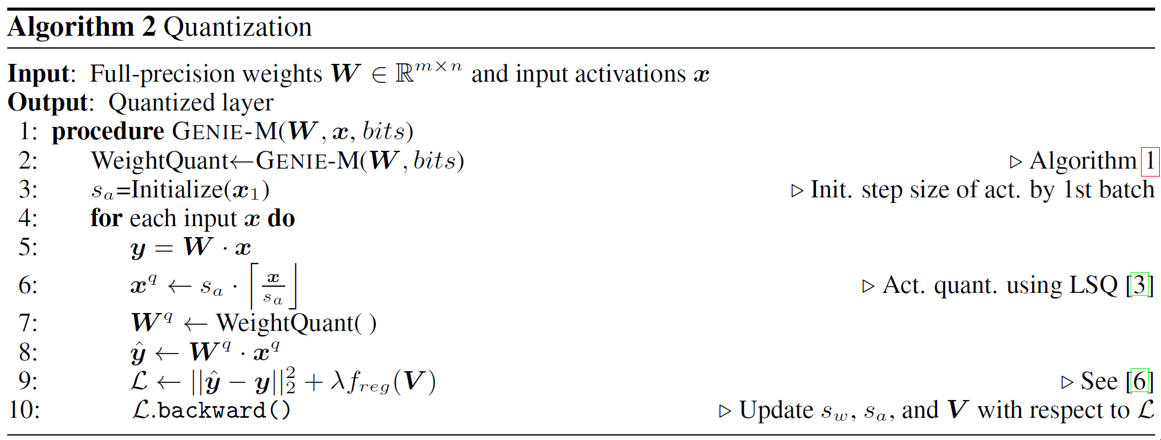## Experimental Results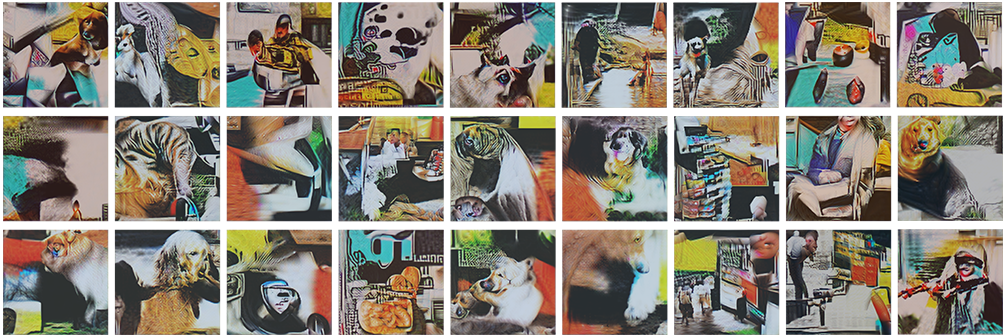Figure 5.   Distilled images by GENIE

Figure 5 shows the distilled images by GENIE without any image prior loss, but they are very similar to the actual structure. Table 1 compares the performance of GENIE with other approaches when bit-width is equal to 2 for weights and 4 for activations. Further details and experimental results can be found in .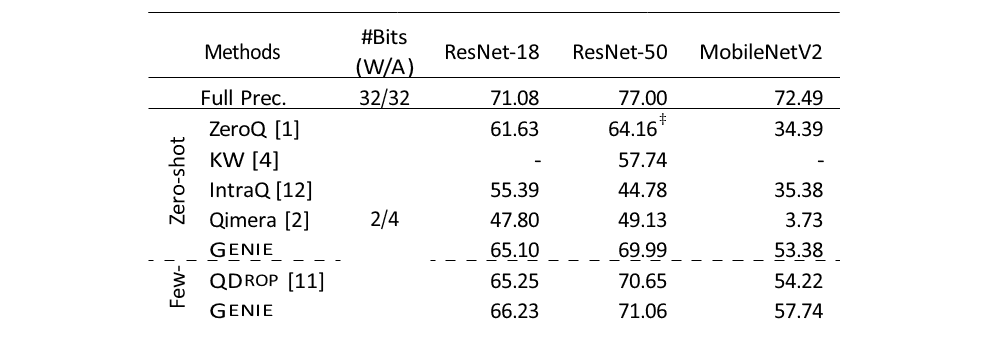Table 1.   Evaluation of CNN Models (top-1 accuracy (%))

In this blog, we have briefly introduced the framework GENIE, which consists of two sub-modules: synthesizing data and quantizing models. GENIE showing outstanding performance compared to existing methods could be a useful framework when deploying AI models on edge devices efficiently.

 About Samsung Research - SoC Architecture Team SoC Architecture Team primarily consists of three parts researching on-device AI such as AI model optimization, AI system software, and AI H/W accelerator. We thus provide the full stack solution for AI on Samsung devices by jointly designing H/W and S/W architectures to meet the performance, cost, and energy requirements, which we call Trinity project. We are sure that our co-design solutions for efficient on-device AI create the unique value of Samsung devices and then offer an extraordinary user experience with the devices. The paper we introduce is related to AI model optimization such that AI models can be deployed on resource-constrained hardware by making AI models be represented to low-bit precision (e.g., INT8) with negligible accuracy drop compared to original FP32 models. These optimized models can be then efficiently processed in AI accelerators on edge devices with the help of AI system software such as compiler and runtime libraries by not having to access cloud servers. This blog is based on and summarizes the paper, "Genie: Show Me the Data for Quantization" which is to appear in CVPR 2023.

https://openaccess.thecvf.com/content/CVPR2023/html/Jeon_Genie_Show_Me_the_Data_for_Quantization_CVPR_2023_paper.html

## References

 Y. Cai, Z. Yao, Z. Dong, A. Gholami, M. W. Mahoney, and K. Keutzer. ZeroQ: A novel zero shot quantization framework. In Proceedings of the IEEE/CVF Conference on Computer Vision and Pattern Recognition (CVPR), pages 13169–13178, 2020.

 K. Choi, D. Hong, N. Park, Y. Kim, and J. Lee. Qimera: Data-free quantization with synthetic boundary supporting samples. Advances in Neural Information Processing Systems (NeurIPS), 34:14835–14847, 2021.

 S. K. Esser, J. L. McKinstry, D. Bablani, R. Appuswamy, and D. S. Modha. Learned step size quantization. In International Conference on Learning Representations (ICLR), 2019.

 J. Gou, B. Yu, S. J. Maybank, and D. Tao. Knowledge distillation: A survey. arXiv preprint arXiv:2006.05525, 2020.

 Y. Jeon, C. Lee, E. Cho, and Y. Ro. Mr. BiQ: Post-training non-uniform quantization based on minimizing the reconstruction error. In Proceedings of the IEEE/CVF Conference on Computer Vision and Pattern Recognition (CVPR), pages 12329–12338, 2022.

 Y. Jeon, C. Lee, and H.-y. Kim. Genie: Show me the data for quantization. arXiv preprint arXiv:2212.04780, 2022.

 Y. Li, R. Gong, X. Tan, Y. Yang, P. Hu, Q. Zhang, F. Yu, W. Wang, and S. Gu. BRECQ: Pushing the limit of post-training quantization by block reconstruction. In International Conference on Learning Representations (ICLR), 2021.

 A. Mordvintsev, C. Olah, and M. Tyka. Deepdream-a code example for visualizing neural networks. Google Research, 2(5), 2015.

 M. Nagel, R. A. Amjad, M. Van Baalen, C. Louizos, and T. Blankevoort. Up or down? adaptive rounding for post-training quantization. In International Conference on Machine Learning (ICML), pages 7197–7206, 2020.

 A. Odena, V. Dumoulin, and C. Olah. Deconvolution and checkerboard artifacts. Distill, 2016. doi: 10.23915/distill.00003. URL http://distill.pub/2016/deconv-checkerboard.

 X. Wei, R. Gong, Y. Li, X. Liu, and F. Yu. QDrop: Randomly dropping quantization for extremely low-bit post-training quantization. In International Conference on Learning Representations (ICLR), 2021.

 Y. Zhong, M. Lin, G. Nan, J. Liu, B. Zhang, Y. Tian, and R. Ji. Intraq: Learning synthetic images with intra-class heterogeneity for zero-shot network quantization. In Proceedings of the IEEE/CVF Conference on Computer Vision and Pattern Recognition (CVPR), pages 12339–12348, 2022.